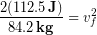# 10.6: Jumping

$$\newcommand{\vecs}{\overset { \rightharpoonup} {\mathbf{#1}} }$$ $$\newcommand{\vecd}{\overset{-\!-\!\rightharpoonup}{\vphantom{a}\smash {#1}}}$$$$\newcommand{\id}{\mathrm{id}}$$ $$\newcommand{\Span}{\mathrm{span}}$$ $$\newcommand{\kernel}{\mathrm{null}\,}$$ $$\newcommand{\range}{\mathrm{range}\,}$$ $$\newcommand{\RealPart}{\mathrm{Re}}$$ $$\newcommand{\ImaginaryPart}{\mathrm{Im}}$$ $$\newcommand{\Argument}{\mathrm{Arg}}$$ $$\newcommand{\norm}{\| #1 \|}$$ $$\newcommand{\inner}{\langle #1, #2 \rangle}$$ $$\newcommand{\Span}{\mathrm{span}}$$ $$\newcommand{\id}{\mathrm{id}}$$ $$\newcommand{\Span}{\mathrm{span}}$$ $$\newcommand{\kernel}{\mathrm{null}\,}$$ $$\newcommand{\range}{\mathrm{range}\,}$$ $$\newcommand{\RealPart}{\mathrm{Re}}$$ $$\newcommand{\ImaginaryPart}{\mathrm{Im}}$$ $$\newcommand{\Argument}{\mathrm{Arg}}$$ $$\newcommand{\norm}{\| #1 \|}$$ $$\newcommand{\inner}{\langle #1, #2 \rangle}$$ $$\newcommand{\Span}{\mathrm{span}}$$$$\newcommand{\AA}{\unicode[.8,0]{x212B}}$$

## Work-Energy Principle

How do we calculate the total work when more than one force acts on an object as it moves, so that each force is doing work? What if the forces point in opposite directions so one does positive work and the other does negative work? In this case we calculate the net work done by each force and add them up (keeping negative works as negative) to get the net work. Alternatively, add up the forces, including directions, to find the size and direction of the net force and then multiply by the distance over which the net force is applied to get the net work. Either way will give you the same answer, which will be the net work. The net work tells us how much energy is transferred into or out of the kinetic energy, causing a change in kinetic energy (). Everything we have discussed so far can be summed up by the work-energy principle:

(1)Alternatively,

(2)or even more explicitly:

(3)#### Everyday Examples: Jumping

During a jump a person’s legs might apply a force of 1200 N upward on their center of mass while the center of mass moves 0.3 m upward. Let’s figure out what their launch velocity and hang-time will be if the person has a weight of 825 N.

First we calculate the work done by their legs.Gravity was acting on them during the launch phase as well, so we need to calculate the work done by gravity, which acts in the opposite direction to motion (\$\theta = 180):Adding up the works to get the net work:The work-energy principle tells us to set the change in kinetic energy equal to the net work. We will keep in mind that they started at rest, so the initial kinetic energy was zero.We isolate the final velocity at the end of the launch phase (as the person leaves the ground)We can see that we need the persons mass. We just need to divide their weight by g = 9.8 m/s/s to find it:We insert the mass:Finally we take the square root of the result to find the final velocity:#### Reinforcement Exercises

When the person in the previous example reaches peak height their instantaneous velocity will be instantaneously zero just as they change direction, so their kinetic energy is also zero at this point.

What was the change in kinetic energy of the person from launch to peak of their jump?

While in the air, the only force acting on them was gravity. The work done by gravity must have caused the change in kinetic energy you found above. That work was done by gravity applied over the distance that they rose through the air. Apply the work-energy principle to the in-air section of the jump to determine the peak height of their jump. [Hint: You already know the size of the force of gravity, it’s their weight of 825 N]

This page titled 10.6: Jumping is shared under a CC BY-NC-SA 4.0 license and was authored, remixed, and/or curated by Lawrence Davis (OpenOregon) via source content that was edited to the style and standards of the LibreTexts platform; a detailed edit history is available upon request.